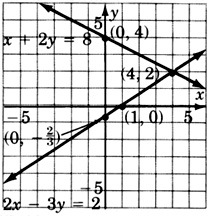# 11.8: Proficiency Exam

$$\newcommand{\vecs}{\overset { \rightharpoonup} {\mathbf{#1}} }$$ $$\newcommand{\vecd}{\overset{-\!-\!\rightharpoonup}{\vphantom{a}\smash {#1}}}$$$$\newcommand{\id}{\mathrm{id}}$$ $$\newcommand{\Span}{\mathrm{span}}$$ $$\newcommand{\kernel}{\mathrm{null}\,}$$ $$\newcommand{\range}{\mathrm{range}\,}$$ $$\newcommand{\RealPart}{\mathrm{Re}}$$ $$\newcommand{\ImaginaryPart}{\mathrm{Im}}$$ $$\newcommand{\Argument}{\mathrm{Arg}}$$ $$\newcommand{\norm}{\| #1 \|}$$ $$\newcommand{\inner}{\langle #1, #2 \rangle}$$ $$\newcommand{\Span}{\mathrm{span}}$$ $$\newcommand{\id}{\mathrm{id}}$$ $$\newcommand{\Span}{\mathrm{span}}$$ $$\newcommand{\kernel}{\mathrm{null}\,}$$ $$\newcommand{\range}{\mathrm{range}\,}$$ $$\newcommand{\RealPart}{\mathrm{Re}}$$ $$\newcommand{\ImaginaryPart}{\mathrm{Im}}$$ $$\newcommand{\Argument}{\mathrm{Arg}}$$ $$\newcommand{\norm}{\| #1 \|}$$ $$\newcommand{\inner}{\langle #1, #2 \rangle}$$ $$\newcommand{\Span}{\mathrm{span}}$$$$\newcommand{\AA}{\unicode[.8,0]{x212B}}$$

## Proficiency Exam

##### Exercise $$\PageIndex{1}$$

Solve using graphing:

$$\left\{\begin{array}{r} 3x + 2y = 4\\ 15x + 10y = -10 \end{array}\right.$$inconsistent##### Exercise $$\PageIndex{2}$$

Solve using graphing:

$$\left\{\begin{array}{r} 2x - 3y = 2\\ x + 2y = 8 \end{array}\right.$$(4,2)##### Exercise $$\PageIndex{3}$$

Solve using substitution:

$$\left\{\begin{array}{r} 2x + 6y = 16\\ x - 4y = -13 \end{array}\right.$$

$$(−1,3)$$

##### Exercise $$\PageIndex{4}$$

$$\left\{\begin{array}{r} 3x + 8y = -5\\ x - 2y = 3 \end{array}\right.$$

$$(1, -1)$$

##### Exercise $$\PageIndex{5}$$

Solve using either substitution or addition:

$$\left\{\begin{array}{r} 4x - 4y = 8\\ x - y = 5 \end{array}\right.$$

inconsistent

##### Exercise $$\PageIndex{6}$$

Solve using either substitution or addition:

$$\left\{\begin{array}{r} 9x + 3y = 12\\ 3x - y = 4 \end{array}\right.$$

$$(\dfrac{4}{3}, 0)$$

##### Exercise $$\PageIndex{7}$$

The sum of two numbers is 43 and the difference of the same two numbers is 7. What are the numbers?

18 and 25

##### Exercise $$\PageIndex{8}$$

A chemist needs 80 ml of an 18% acid solution. She has two acid solutions, A and B, to mix together to form the 80-ml solution. Acid solution A is 15% acid and acid solution B is 20% acid. How much of each solution should be used?

32 ml of solution A;  48 ml of solution B.

A parking meter contains 32 coins. If the meter contains only nickels and quarters, and the total value of the coins is $4.60, how many of each type of coin are there? Answer 17 nickels and 15 quarters ##### Exercise $$\PageIndex{10}$$ A person has$15,000 to invest. If he invests part at 8% and the rest at 12%, how much should he invest at each rate to produce the same return as if he had invested it all at 9%?

$11,250 at 8%;$3,750 at 12%